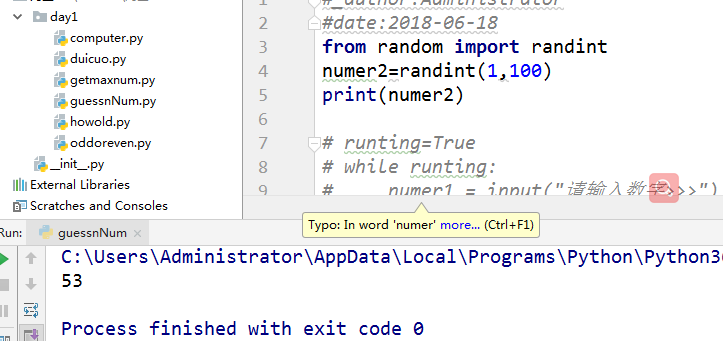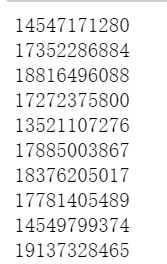• python中经常会需要随机生成一些数字，此时就需要用到python中的random模块中的randint函数了，方法如图：此时执行程序时会随机生成闭区间1到100之间的数字，包含1和100，但这里需求注意的是，random.randint(a, b...
在python中经常会需要随机生成一些数字，此时就需要用到python中的random模块中的randint函数了，方法如图：此时执行程序时会随机生成闭区间1到100之间的数字，包含1和100，
但这里需求注意的是，
random.randint(a, b)     # 返回闭区间 [a, b] 范围内的整数值
numpy.random.randint(a, b)   # 返回开区间 [a, b) 范围内的整数值
在使用时需要区分、注意
展开全文• Python语言学习：利用Python随机生成那些事之随机生成使用方法、案例应用之详细攻略 目录 案例应用 1、随机生成指定区间内的某一值 案例应用 1、随机生成指定区间内的某一值 使用说明：给定区域...
Python语言学习：利用Python随机生成那些事之随机生成使用方法、案例应用之详细攻略

目录
案例应用
1、随机生成指定区间内的某一值

案例应用
1、随机生成指定区间内的某一值
使用说明：给定区域范围，随机输出该范围内任意数值
import numpy as np

def r(val):    #r()函数：返回输入值的百分比的整数，即返回值为整数，且一定≤输入值
return int(np.random.random() * val)

def rand_range(lo,hi):                      #rand_range()函数
return lo+r(hi-lo);

print(rand_range(0,31))      #随机输出区间值
print(rand_range(31,65))     #随机输出区间值

11
37


展开全文• 使用python随机函数随机批量生成手机号和姓名 ** 利用random函数随机生成姓名和手机号，用到的函数主要有random.randint、random.choice、“sep”.join(seq)，要改生成的名字、手机号的数量只需要改range()里面的...
**
使用python随机函数随机批量生成手机号和姓名
**
利用random函数随机生成姓名和手机号，用到的函数主要有random.randint、random.choice、“sep”.join(seq)，要改生成的名字、手机号的数量只需要改range()里面的数值就行。
随机生成名字的第一种写法：
#第一种写法
import random
for i in range(1000):
def name():
xing=random.choice(["赵","钱","孙","李","周","吴"])
ming="".join(random.choice(["义","凝","好","九","立","风","离",""])for i in range(1,4))
return xing+ming
newname=name()
print(newname)


结果如下：随机生成名字的第二种写法：
#第二种写法
for i in range(10):
xing='赵钱孙李周吴郑王'
ming='人间四月芳菲尽山寺桃花始胜开'
x=random.choice(xing)
m="".join(random.choice(ming) for i in range(1,3))
x+m
print(x+m)随机生成名字的第三种写法，这种写法有点累赘：
#第三种写法
def name():
xing=random.choice(["赵","钱","孙","李","周","吴"])
ming1=random.choice(["义","凝","好","九","立","风","离",""])
ming2=random.choice(["家","月","集","必","","楼","音"])
return xing+ming1+ming2

for i in range(10):
newname=name()
print(newname)批量随机生成手机号，注释掉的是另一种随机数的取法。
def phone():
#创建手机号第二位
second=random.choice([3,4,5,7,8,9]) #从这个list里面随机选择一个数字
#创建手机号第三位
third={
3:random.randint(0,9),      #从0-9之间随机生成一个整数int类型
4:random.choice([5,7,9]),
#4:[5,7,9][random.randint(0,2)],
5:random.choice([0,1,2,3,4,5,7,8]),
#5:[i for i in range(10) if i !=4][random.randint(0,8)],
7:random.choice([2,3,5,6,7,8]),
#7:[i for i in range(10) if i not in [4,9]][random.randint(0,7)],
8:random.randint(0,9),
9:random.choice([1,3,8,9]),
#9:[1,3,8,9][random.randint(0,2)],
}[second]  #third后面加上second是由于third的生成要依赖second的随机选择结果
#创建手机号最后八位
suffix=random.randint(9999999,100000000)
#拼接手机号
return "1{}{}{}".format(second,third,suffix)

#创建批量生成手机号的循环
for i in range(10):
randomphone=phone()
print(randomphone)有不严谨的地方请多指正。
展开全文数据分析 random
• Python随机生成体温并写入Excel 疫情期间在校需要时常测量体温，早晚各一次，并且要填入Excel表格中汇总，十分麻烦，所以写了一个Python程序生成随机数作为温度填入Excel中。 # coding=UTF-8 import random import ...
Python随机生成体温并写入Excel
疫情期间在校需要时常测量体温，早晚各一次，并且要填入Excel表格中汇总，十分麻烦，所以写了一个Python程序生成随机数作为温度填入Excel中。

直接用代码之前要新建xls文件，注意别新建成xlsx了！

# coding=UTF-8
import random
import xlrd
import xlwt
from xlutils.copy import copy
# 前几列需要一些基本信息  用此函数生成
def create_value():
date=1  # 生成一天的数据
all_value=[]
first_row = ['学号','姓名']
second_row = ['','']
stu1=['xxxxxxxxxx','张三']
stu2=['xxxxxxxxxx','李四']
stu3=['xxxxxxxxxx','王五']
stu4=['xxxxxxxxxx','王六']
stu5=['xxxxxxxxxx','王七']
stu6=['xxxxxxxxxx','何八']
stu7=['xxxxxxxxxx','王二']
for i in range(date):
first_row.append('6月%d日'%(i+1))
first_row.append('')
second_row.append('上午温度')
second_row.append('下午温度')
for i in range(date*2):
stu1.append('36.%d' % random.randint(0, 9))
stu2.append('36.%d' % random.randint(0, 9))
stu3.append('36.%d' % random.randint(0, 9))
stu4.append('36.%d' % random.randint(0, 9))
stu5.append('36.%d' % random.randint(0, 9))
stu6.append('36.%d' % random.randint(0, 9))
stu7.append('36.%d' % random.randint(0, 9))
all_value.append(first_row )
all_value.append(second_row )
all_value.append(stu1)
all_value.append(stu2)
all_value.append(stu3)
all_value.append(stu4)
all_value.append(stu5)
all_value.append(stu6)
all_value.append(stu7)
return all_value

def write_excel_xls(path, sheet_name, value):
index = len(value)  # 获取需要写入数据的行数
workbook = xlwt.Workbook()  # 新建一个工作簿
for i in range(0, index):
for j in range(0, len(value[i])):
sheet.write(i, j, value[i][j])  # 像表格中写入数据（对应的行和列）
workbook.save(path)  # 保存工作簿
print("xls格式表格写入数据成功！")

# 列追加数据
def write_excel_xls_append(path, value):
index = len(value)  # 获取需要写入数据的行数
workbook = xlrd.open_workbook(path)  # 打开工作簿
sheets = workbook.sheet_names()  # 获取工作簿中的所有表格
worksheet = workbook.sheet_by_name(sheets)  # 获取工作簿中所有表格中的的第一个表格
cols_old = worksheet.ncols  # 获取表格中已存在的数据的列数
new_workbook = copy(workbook)  # 将xlrd对象拷贝转化为xlwt对象
new_worksheet = new_workbook.get_sheet(0)  # 获取转化后工作簿中的第一个表格
for i in range(0, index):
for j in range(0, len(value[i])):
new_worksheet.write(i, j+cols_old, value[i][j])  # 追加写入数据
new_workbook.save(path)  # 保存工作簿
print("xls格式表格【追加】写入数据成功！")
# 此处是行追加数据#####
# def write_excel_xls_append(path, value):
#     index = len(value)  # 获取需要写入数据的列数
#     workbook = xlrd.open_workbook(path)  # 打开工作簿
#     sheets = workbook.sheet_names()  # 获取工作簿中的所有表格
#     worksheet = workbook.sheet_by_name(sheets)  # 获取工作簿中所有表格中的的第一个表格
#     rows_old = worksheet.nrows  # 获取表格中已存在的数据的行数
#     new_workbook = copy(workbook)  # 将xlrd对象拷贝转化为xlwt对象
#     new_worksheet = new_workbook.get_sheet(0)  # 获取转化后工作簿中的第一个表格
#     for i in range(0, index):
#         for j in range(0, len(value[i])):
#             new_worksheet.write(i + rows_old, j, value[i][j])  # 追加写入数据，注意是从i+rows_old行开始写入
#     new_workbook.save(path)  # 保存工作簿
#     print("xls格式表格【追加】写入数据成功！")

workbook = xlrd.open_workbook(path)  # 打开工作簿
sheets = workbook.sheet_names()  # 获取工作簿中的所有表格
worksheet = workbook.sheet_by_name(sheets)  # 获取工作簿中所有表格中的的第一个表格
for i in range(0, worksheet.nrows):
for j in range(0, worksheet.ncols):
print(worksheet.cell_value(i, j), "\t", end="")  # 逐行逐列读取数据
print()

date=1
all_value=[]
first_row = []
second_row = []
stu1=[]
stu2=[]
stu3=[]
stu4=[]
stu5=[]
stu6=[]
stu7=[]
index = len(value)  # 获取需要写入数据的行数
workbook = xlrd.open_workbook(path)  # 打开工作簿
sheets = workbook.sheet_names()  # 获取工作簿中的所有表格
worksheet = workbook.sheet_by_name(sheets)  # 获取工作簿中所有表格中的的第一个表格
cols_old = worksheet.ncols  # 获取表格中已存在的数据的列数
first_row.append('6月%d日'%((cols_old-2)/2+1))
first_row.append('')
second_row.append('上午温度')
second_row.append('下午温度')
for i in range(date*2):
stu1.append('36.%d' % random.randint(0, 9))
stu2.append('36.%d' % random.randint(0, 9))
stu3.append('36.%d' % random.randint(0, 9))
stu4.append('36.%d' % random.randint(0, 9))
stu5.append('36.%d' % random.randint(0, 9))
stu6.append('36.%d' % random.randint(0, 9))
stu7.append('36.%d' % random.randint(0, 9))
all_value.append(first_row)
all_value.append(second_row)
all_value.append(stu1)
all_value.append(stu2)
all_value.append(stu3)
all_value.append(stu4)
all_value.append(stu5)
all_value.append(stu6)
all_value.append(stu7)
return all_value

sheet_name_xls = '6月温度'
value = create_value() # 第一天记录时需要写入基本信息，用此数据写入

展开全文• 今天小编就为大家分享一篇python随机生成库faker库api实例详解，具有很好的参考价值，希望对大家有所帮助。一起跟随小编过来看看吧
• 所以这事不适合人干，但机器最做这个最拿手，今天我们就来学习一段python脚本，可以直接生成自己需要的密码，且密码长度，数字，字符个数随意你指定，废话不多说了，我们直接看代码： #!/usr/bin/env python import...
• Python有一随机函数可以产生[0,1)区间内的随机数，基于此函数生成随机分布在任意三角形内的点 由数学知识得知： 几何体的向量表达形式 直线： 线段： 推广到高维 三维平面： ...Python随机生成
• def random_number(num2): list2 = [] for number in range(num2): str2 = str(random.randint(0, 9)) list2.append(str2) ...随机生成1位随机数 random_number(5) 结果 94837 随机生成5位随机数random
• python 随机生成大乐透号码 自己写来买彩票用的，如下： 自己写来买彩票用的，如下： import random L1 = [ i+1 for i in range(35)] L2 = [ i+1 for i in range(12)] def act(N,*arg): res=list(arg) random....
• Python有一随机函数可以产生[0,1)区间内的随机数，但是如果我们想生成随机分布在单位圆上的，那么我们可以首先生成随机分布在单位圆边上的点，然后随机调整每个点距离原点的距离，但是我们发现这个距离不是均匀分布...
• python中常用的随机函数的用法汇总分类以及相似函数之间的区别 1. 常用的随机函数总结介绍 random.random(): 返回随机生成的一个浮点数，范围在[0,1)之间 random.uniform(a, b): 返回随机生成的一个浮点数，范围在...数据挖掘 机器学习
• 随机生成10位数密码，字母和数字组合 import string >>> import random >>> pwd = >>> letters=string.ascii_letters+string.digits >>> for i in range(10): ... letter=random.choice(letters) ... pwd += ...
• Python双色球是一种比较常见的彩票玩法， ...请编写函数随机生成一注双色球彩票，并打印出来，结果是完全随机的。 import random def Red(number,start,end): a = [] while True: a.append(random.randint(s...
• python 随机生成简单语法结构的中文句子 题目如下：Writing a programming which could generate random Chinese sentences based on one grammar. Your input grammar is: simple_grammar = “”" sentence => ...
•  ”’ 随机生成6位的验证码 ”’  code_list = []  for i in range(10): # 0-9数字  code_list.append(str(i))  for i in range(65, 91): # A-Z  code_list.append(chr(i))  for i in range(97, 123): # a-z ...
• Python有一个内建函数叫做random，是用来生成随机数的，但是这个模块存在很大的不足，一次只能生成一个值；而numpy库里的random模块则很好的弥补了它的不足，numpy.random可以高效的生成多种概率分布下的完整样本...random
• 通过Python编写一个成绩统计程序，要求输入成绩表格文件（CSV格式，包括学号、姓名、性别、语文、数学、英语、体育、艺术、技术），程序能够读取一个班的成绩表格文件，进行数据可视化分析。 1. 使用随机数函数产生...flask
• ## Python随机函数

千次阅读 2018-06-27 09:57:49
Python中的random模块用于生成随机数。下面介绍一下random模块中最常用的几个函数。random.randomrandom.random()用于生成一个0到1的随机符点数: 0 &lt;= n &lt; 1.0random.uniformrandom.uniform的函数原型...
• Python随机生成验证码的方法有很多，今天给大家列举两种，大家也可以在这个基础上进行改造，设计出适合自己的验证码方法 方法一： 利用range方法，对于range方法不清楚的同学，请参考文章《python开发的range...
• 今天写代码的时候需要用到随机数，仅仅使用torch.randint(1,10,(4,))发现会出来相同的数。 为了得到不同的随机数，可以利用Python中的random.sample()函数...#表示从[low, high]区间随机生成N个数，结果以列表返回 ...
•概率论与数理统计
• 最近项目中需要自己制造大量数据，并对greenplum的插件--gptext（全文检索）的性能进行测试。如果直接用python进行写入，性能太差，每小时大约插入几十万的...1、使用Python随机生成数据： 具体方法见：https://bl...greenplum copy
• Python Every Day， 第 42 期今天的题目是生成一个8位数字，要求他们的和为40在解这道题之前想先介绍一下random() 函数random()函数：用于...
• 今天学习python是看到...#生成随机测试文件 #第一步将测试数据保存在一个字典中 import random #测试数据 capitals={'印度':'新德里','土耳其':'安卡拉','泰国':'曼谷','缅甸':'仰光',  '意大利':'罗马','葡萄...
• #!/usr/bin/envpython #-*-coding:UTF-8-*- importrandom #生成随机6位随机数含字母 code=[] foriinrange(6): ifi==random.randint(1,5): code.append(str(random.randint(1,5))) el...
• 实验：python批量生成随机信息 这个实验很简单，直接上代码了~~ 一、常用汉字的Unicode编码   在unicode码中,汉字的范围是(0x4e00,0x9fbf)，但是其中包含了很多生僻字或者繁体字，常用汉字的unicode码为： list1 =...信息安全
• 前面已经接触了用turtle模块画图，并且了解了自定义函数的写法，那么根据前面的知识，我们接触下random模块； 首先引入 import random； random.randrange(x):用于生成0-x之间的随机数； random.randrange(x,y):...
• python中的numpy.random.randint(a,b)用于生成一个指定范围内的c行d列的整数矩阵。其中参数a是下限，参数b是上限，生成的随机数矩阵n: a <= n <= b。前提是必须import numpy，如果直接import random后调用...txt存储...

# python随机生成数字的函数python 订阅